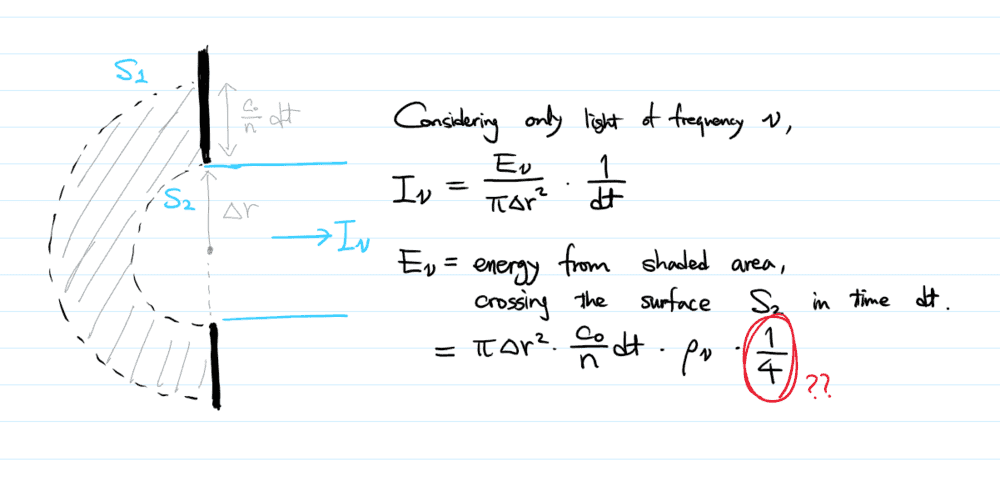# Relation between spectral intensity and spectral energy density

• I
IcedCoffee
TL;DR Summary
How is the following relation between spectral intensity and spectral energy density derived?
In Principles of Lasers by Svelto, while deriving the Planck radiation formula, equation 2.2.3 says $$I_{\nu} = \frac {c_0} {4n} \rho_\nu$$
where ##I_\nu## is the spectral intensity at some hole in the cavity wall (energy per time per area per frequency),
##c_0## is the speed of light in vacuum,
##n## is the refractive index of the medium inside the cavity,
and ##\rho_\nu## is the spectral energy density inside the cavity (energy per volume per frequency).

I understand that in the case of monochromatic wave propagating in one direction, ##I = \frac {c_0} {n} \rho##
since multiplying both sides by ##dt## would give the amount of energy passing through a given area (perpendicular to the direction of propagation),
which must originate from the volume filled with the energy density ##\rho##.

However, in this case, where does the factor of ## \frac 1 4## come from?